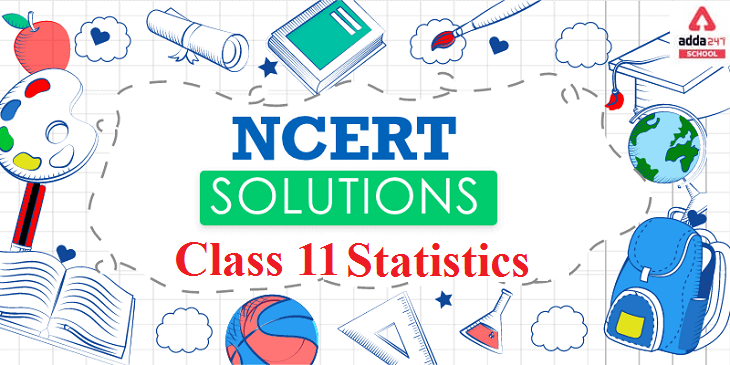Online Tution   »   Ncert Solution Class 11 Statistics

# NCERT Solutions For Statistics Class 11NCERT Solutions For Statistics Class 11: NCERT Solutions provides an overall overview of the units and the chapters covered within each unit. Here, we will provide the complete information about the revised and updated syllabus.

The revised syllabus of Statistics subject of class 11 involves various topics and concepts that prepares students for better future with clear base of the subject.

Statistics is a mathematical body of science that deals with the collection, analysis, interpretation, presentation of numerical data and masses. In other word Statistics is a group of quantitative data. The word “Statistics” is often used, it is derived from the Latin word ‘Status’ which means a collection of figures or numbers; those constitute some data of our human interest.

The NCERT Solutions PDFs at ADDA 247 provides a accurate and easy solution for all the problems covered in the NCERT books. ADDA 247 develop a solid conceptual base in students which plays a significant role in the latter stages of preparing for the competitive exams. We provide detailed solution to NCERT problems with a prescribed syllabus.

### NCERT Solutions For Class 11 Statistics Chapterwise PDF

NCERT solutions for Class 11 Statistics, chapter wise is given below.

 Units Name of the Chapter Chapter 1 Introduction Chapter 2 Collection of Data Chapter 3 Organisation of Data Chapter 4 Presentation of Data Chapter 5 Measures of Central Tendency Chapter 6 Measures of Dispersion Chapter 7 Correlation Chapter 8 Index Numbers Chapter 9 Use of Statistical Tools

## NCERT Solutions Class 11 Statistics: Syllabus

 SYLLABUS OF STATISTICS CLASS-11 CHAPTER NO. NAME OF THE CHAPTER MARKS Chapter-1. Introduction Chapter-2. Collection of Data Chapter-3. Organization of Data Chapter-4. Presentation of Data Chapter-5. Measures of Central Tendency Chapter-6. Measures of Dispersion Chapter-7. Correlation Chapter-8. Index Numbers Chapter-9. Use of Statistical Tools

CHAPTER-1: What is Economics, Meaning, Scope and Importance of Statistics in Economics

CHAPTER-2: Collection of data, Source of data- Primary and Secondary data, how basic data is collected, Method of collection of data, Some important source of secondary data, Census of India and national sample survey organization

CHAPTER-3: Meaning and types of variables, Frequency distribution

CHAPTER-4: Tabular Presentation and Diagrammatic Presentation of Data: 1. Geometric forms (Bar Diagram and Pie Diagram), 2. Frequency Diagram (Histogram, Polygon and Ogive), 3. Arithmetic line Graphs (Time Series Graph)

CHAPTER-5: Mean (Simple and Weighted), Median and Mode

CHAPTER-6: Absolute Dispersion (Range, Quartile Deviation, Mean Deviation, Standard Deviation), Relative Dispersion (Co-Efficient of Quartile Deviation, Co-Efficient of Mean Deviation, Co-Efficient of Variation), Lorenz curve, Meaning and its Application

CHAPTER-7: Meaning, Scatter Diagram, Measures of Correlation, Karl Persons Method, Spearman’s Rank Correlation

CHAPTER-8: Meaning, Types- Wholesale Price Index, Consumer Price Index and Index of Industrial Production, Use of Index Numbers, Inflation and Index Numbers

## FAQs for NCERT Solutions Class 11 Statistics

Q1. Which are the important topics for class 11 statistics?

ANS. The important topics for class 11 statistics that the students must study in detail are meaning of economy, meaning of statistics, Indian economy, correlation, dispersion, central tendency, collection of data, presentation and organization of data, Indian economy-1950-1990, liberalization, privatization, globalization, employment, infrastructure, sustainable development, rural development etc.

Q2. What is meant by Sustainable development?

ANS. The concept of sustainable development is a critical notion that is meant to define the process by which a country manages its production without causing depletion in the country’s natural resources and bringing about environmental degradation.

Q3. How can I score 100 marks in Statistics in CBSE class 11?

ANS. You can score full marks in statistics by doing practice daily and follow as per revised syllabus of Economics and for more information visit Adda 247.

Q4. How helpful is NCERT statistics class 11 Solutions?

ANS. The NCERT Solution for class 11 is extremely helpful for students preparing for the board examination. The solution provides a detailed definition of crucial economic concepts like data collection, central tendency, sustainable development etc. so that the student can effectively learn the basics.

The solution also helps students to understand the concepts and score high marks and also strengthen students understanding of fundamental concepts on the topic. The language of the solution is kept lucid so that students have no problem understanding it.